## 1. 前言

• 单链表：结点中存储数据和其后驱结点的地址。
• 循环链表：在单链表的基础上，其最后一个结点，也称尾结点中存储头结点的地址，形成一个闭环。
• 双向链表：结点中存储数据和前、后驱结点的地址。
• 双向循环链表：在双向链表的基础上，头结点保存尾结点地址，尾结点保存头结点地址。一般说双向链表都是指双向循环链表。

## 2. 单链表

• 存放数据信息，称为数据域。
• 存放后驱结点的地址信息，称为指针域。`C++`中可以使用结构体描述结点：

``````typedef int dataType;
//结点
//数据成员
dataType  data;
//后驱结点的地址
//构造函数
this->data=data;
this->next=NULL;
}
};
``````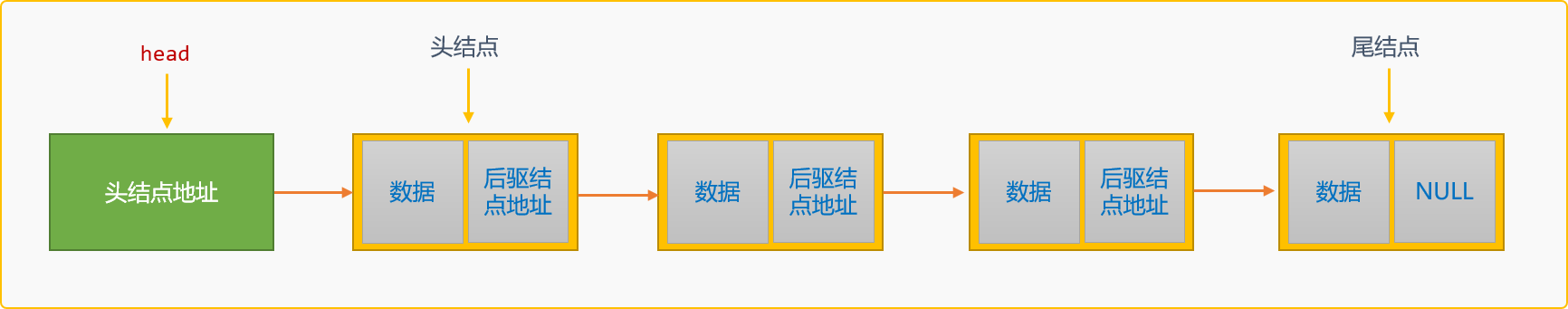`链表``2` 个特殊结点：

• 头结点：链表的第一个结点，头结点没有前驱结点。头结点可以存储数据，也可以不存储数据，不存储时，此结点为标识用的空白结点，可在链表操作时提供便利。关于第一结点的问题在后文会详细介绍。
• 尾结点：链表中的最后一个结点，在单链表中其后驱结点的地址为`NULL`，也就是没有后驱结点

``````/*
* 链表类
*/
private:
//头指针
//链表的长度
int length;
public:
//构造函数
//返回头指针
}
//初始化链表
//按位置查询结点
LinkNode * findNodeByIndex(int index) {}
//从头创建链表
void createFromHead(int n) {}
//从尾建链表
void createFromTail(int n) {}
//按值查询结点
LinkNode * findNodeByVal(dataType val) {}
//后插入
int instertAfter(dataType val,dataType data) {}
//前插入
int insertBefore(dataType val,dataType data) {}
//按位置删除结点
int delNode(dataType data) {}
//删除所有结点
void delAll() {}
//显示所有
void showSelf() {}
//析构函数
this->delAll();
}
};
``````

### 2.1 初始化

`head``NULL`时，说明此链表为空链表。一般会在初始化时，为`head`变量存储一个没有实际数据语义的标志性结点，也称为空白头结点。

• 设置 `head``NULL`。建立一个空链表。``````//初始化链表
}
``````
• 设置`head`指向一个没有实际数据语义的空白结点，此结点仅起到标志作用。``````//初始化链表
}
``````

### 2.2 创建单链表

• 创建过程中，新结点替换原来的头结点，成为新的头结点，也称为头部插入创建方案。如构建数据为`{4,9,12,7}`的单链表。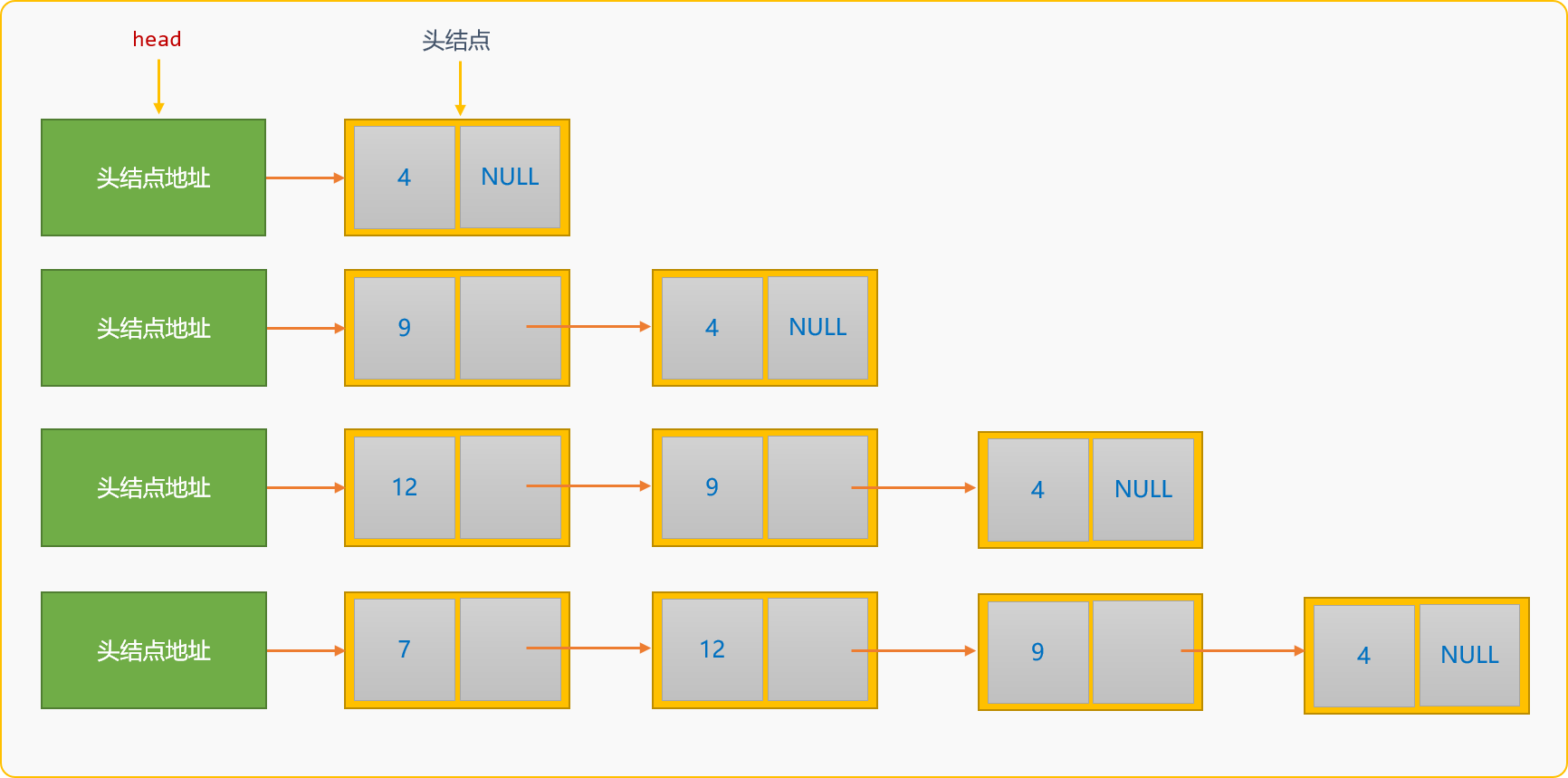``````//头部插入创建链表
void createFromHead(int n) {
for(int i=0; i<n; i++) {
//输入数据
cin>>newNode->data;
//原头结点为新结点的后驱结点
newNode->next=p;
//新结点成为新的头结点
p=newNode;
}
}
``````

``````LinkList list;
cout<<"输出结点信息"<<endl;
//显示第一个结点
//显示第二个结点
//显示第三个结点
``````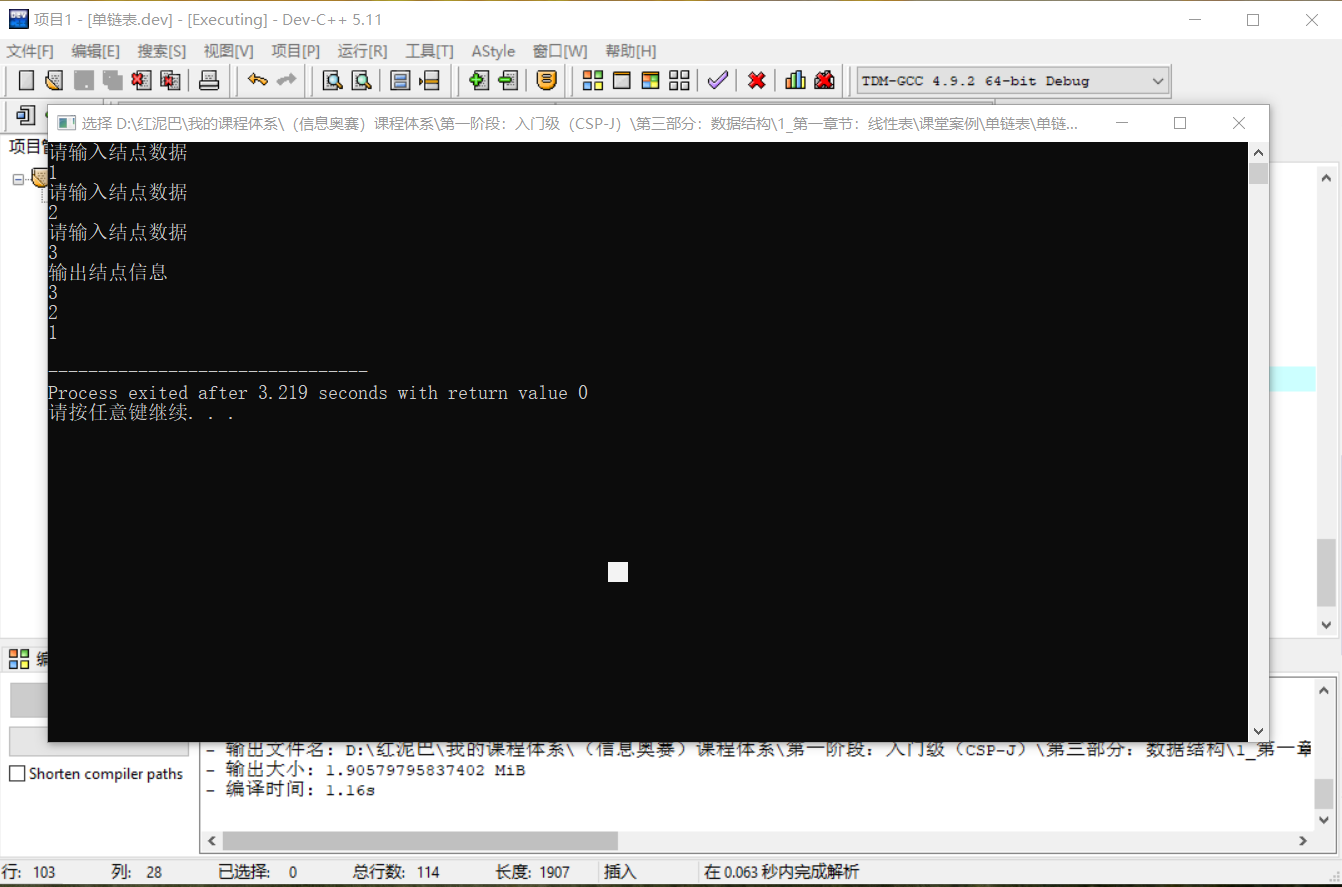• 尾部插入创建单链表，创建时的新结点替换原来的尾结点。如构建数据为`{4,9,12,7}`的单链表。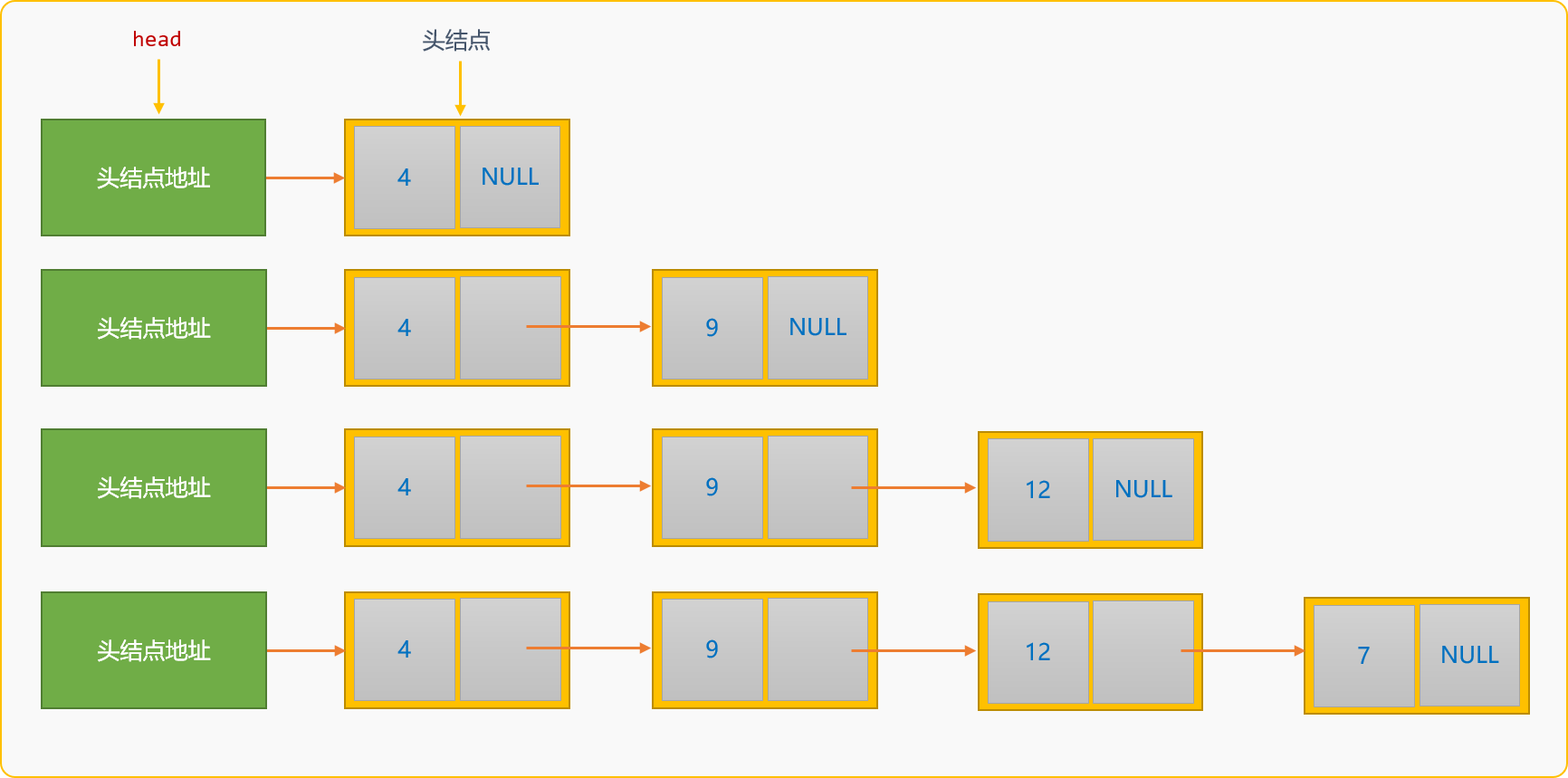``````//从尾建链表
void createFromTail(int n) {
//头结点地址
//尾结点地址
for(int i=0; i<n; i++) {
//构建一个新结点
cout<<"请输入结点数据"<<endl;
cin>>newNode->data;
if(p==NULL) {
//如果头结点为 NULL
p=tail=newNode;
} else {
//原来的尾结点成为新结点的前驱结点
tail->next=newNode;
//新结点成为新的尾结点
tail=newNode;
}
}
}
``````

``````int main(){
list.createFromTail(3);
cout<<"输出结点信息"<<endl;
//显示第一个结点
//显示第二个结点
//显示第三个结点
}
``````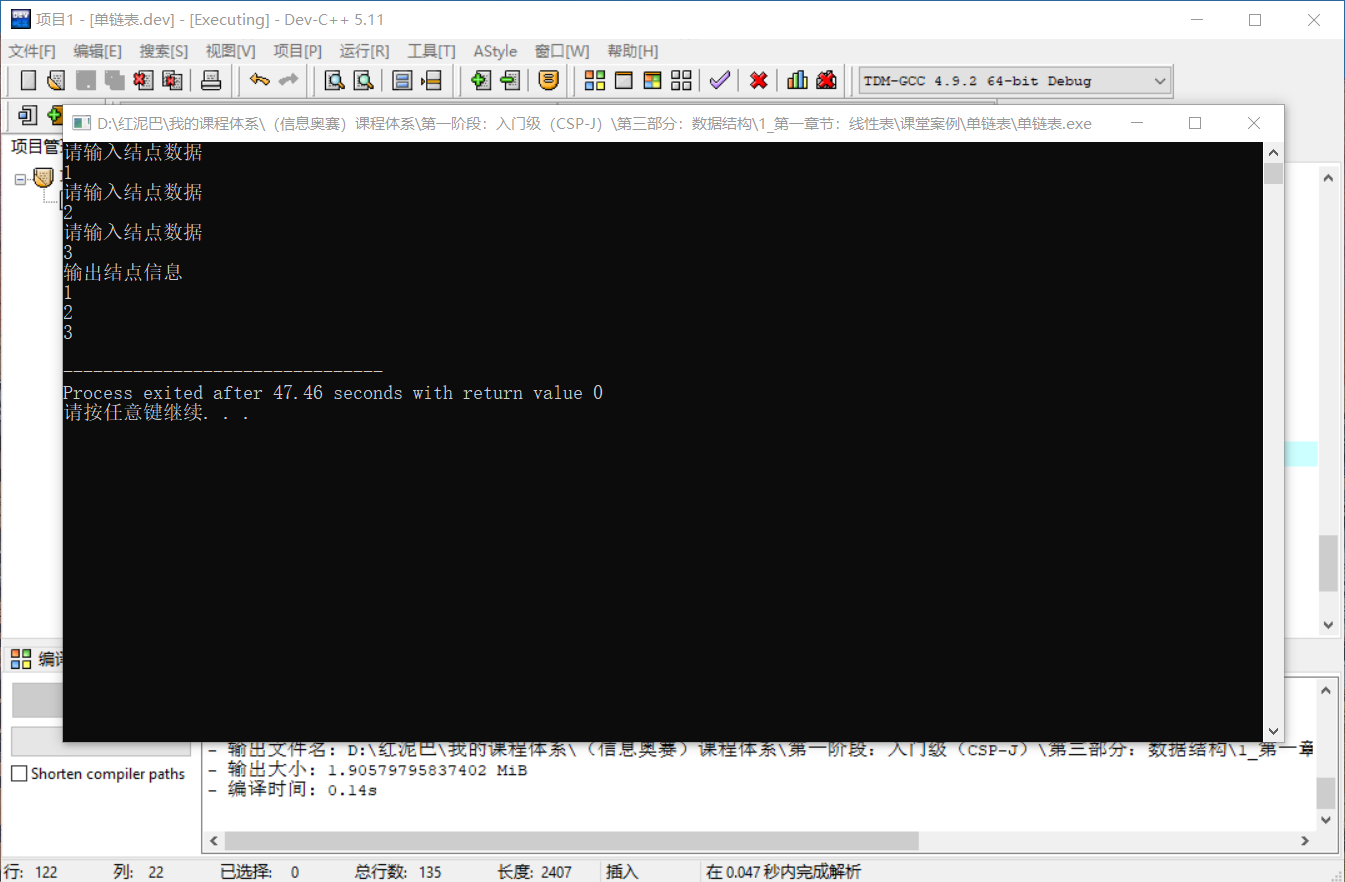``````if(p==NULL) {
//如果头结点为 NULL
p=tail=newNode;
}
``````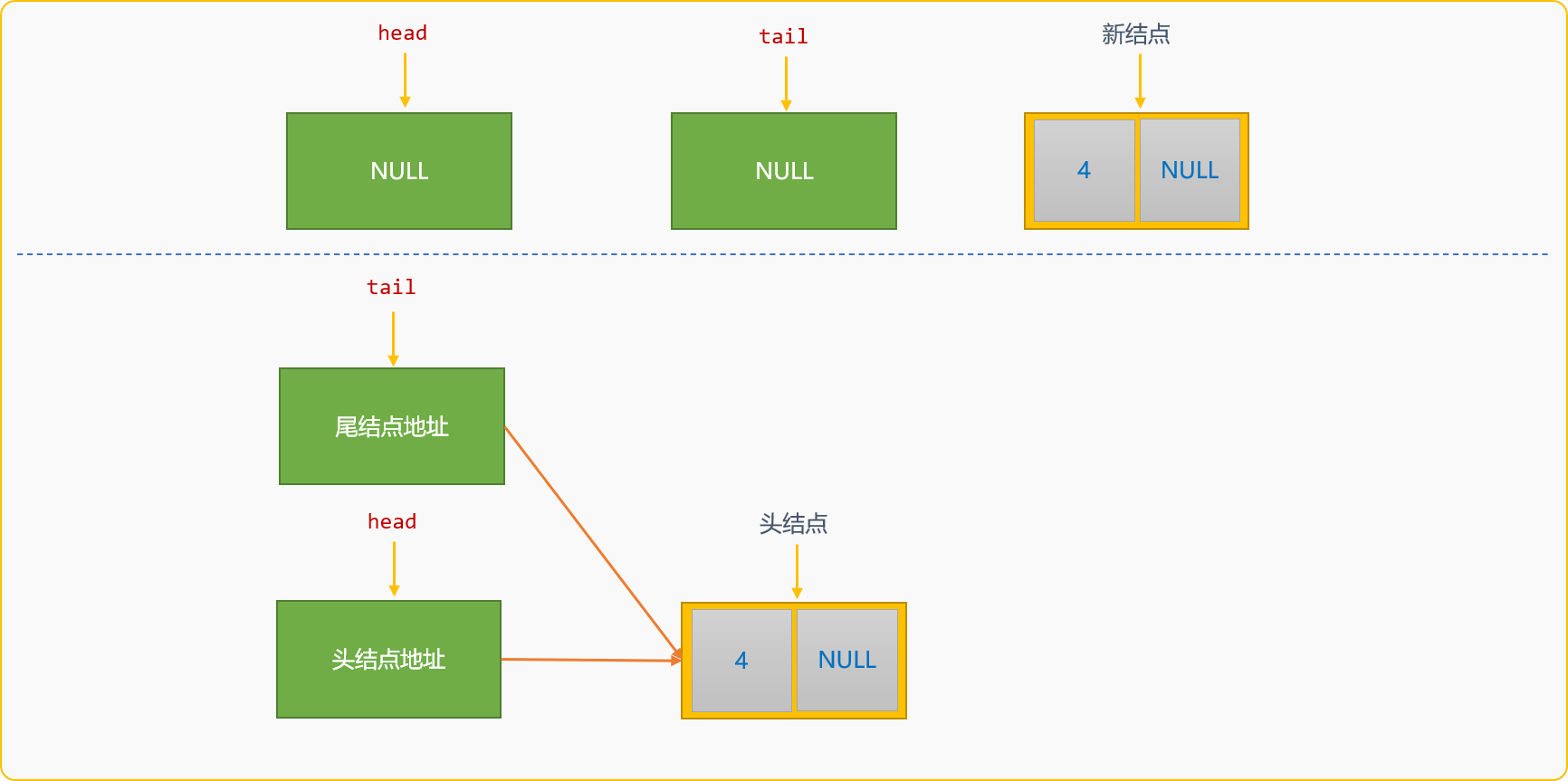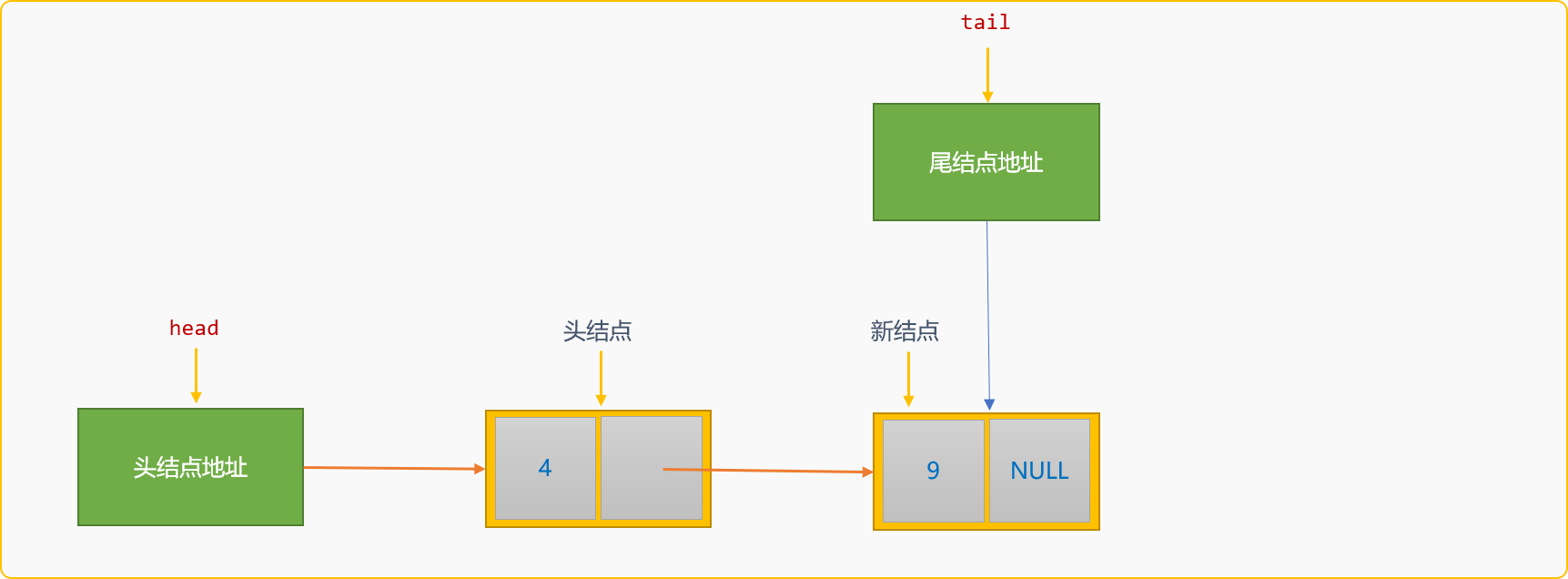``````//初始化链表时指定一个空白结点作为头结点
}
``````

``````void createFromTail_(int n) {
//头结点地址
//尾结点地址
tail=p;
for(int i=0; i<n; i++) {
//构建一个新结点
cout<<"请输入结点数据"<<endl;
cin>>newNode->data;
//原来的尾结点成为新结点的前驱结点
tail->next=newNode;
//新结点成为新的尾结点
tail=newNode;
}
}
``````

### 2.3 查询链表

• 按结点所在位置查找。
• 按结点的值查找。
• 查询链表中的所有结点。

#### 2.3.1 按结点位置查找

``````//按位置查询结点
LinkNode * findNodeByIndex(int index) {
int j=0;
if(index==j)
//如果 index 值为 0 ，返回头结点
return p;
p=p->next;
while( p!=NULL && j<index) {
p=p->next;
j++;
}
if(j==index)return p;
else return NULL;
}
``````

#### 2.3.2 按结点的值查找

``````//按值查询结点
LinkNode * findNodeByVal(dataType val) {
//如果不带空白结点，则从头结点开始查找
whil(p!=null && p->data!=val ) {
p=p->next;
}
if(p!=NULL) {
return p;
} else {
return NULL;
}
}
``````

``````LinkeNode *p=this->head->next;
``````

#### 2.3.3 查询所有

``````//显示所有
void showSelf() {
while(p!=NULL) {
cout<<p->data<<"\t";
p=p->next;
}
}
``````

``````//显示所有
void showSelf() {
while(p!=NULL) {
cout<<p->data<<"\t";
p=p->next;
}
}
``````

### 2.4 插入

• 后插入。
• 前插入。

#### 2.4.1 后插入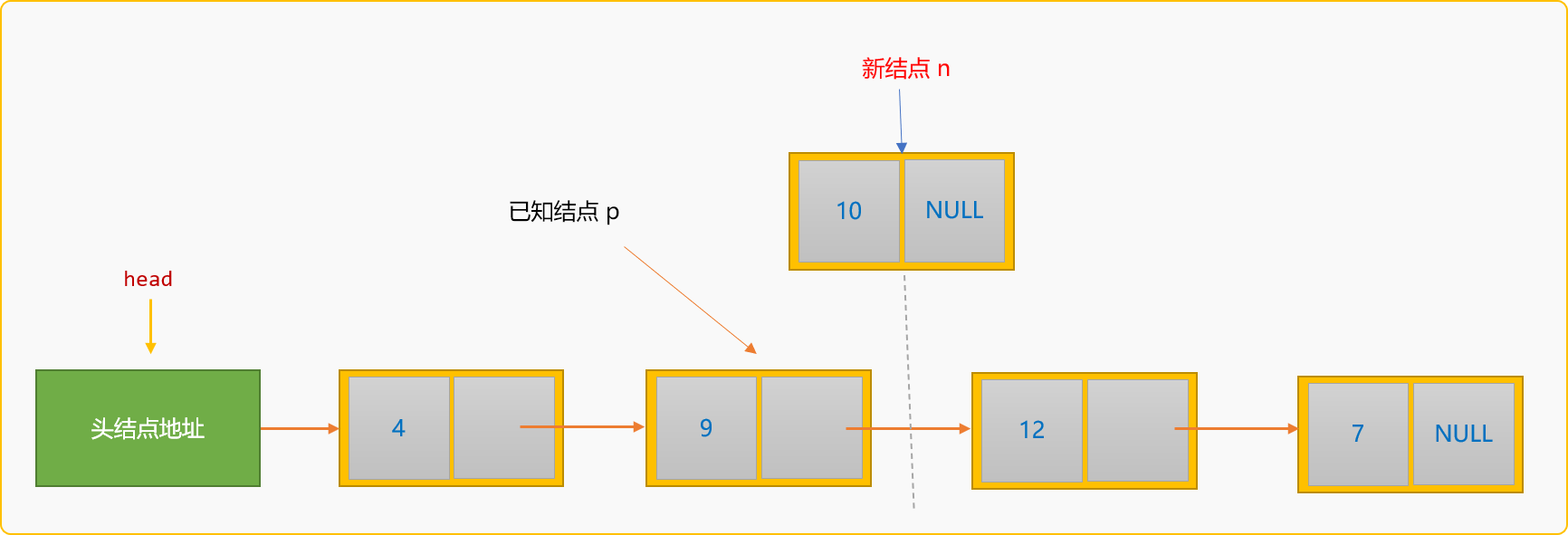• 查找到值为`9`的结点地址，称为 `p`结点。

• 创建新结点。

• 指定新结点`n`的后驱结点为`p`的后驱结点。

``````n->next=p->next;
``````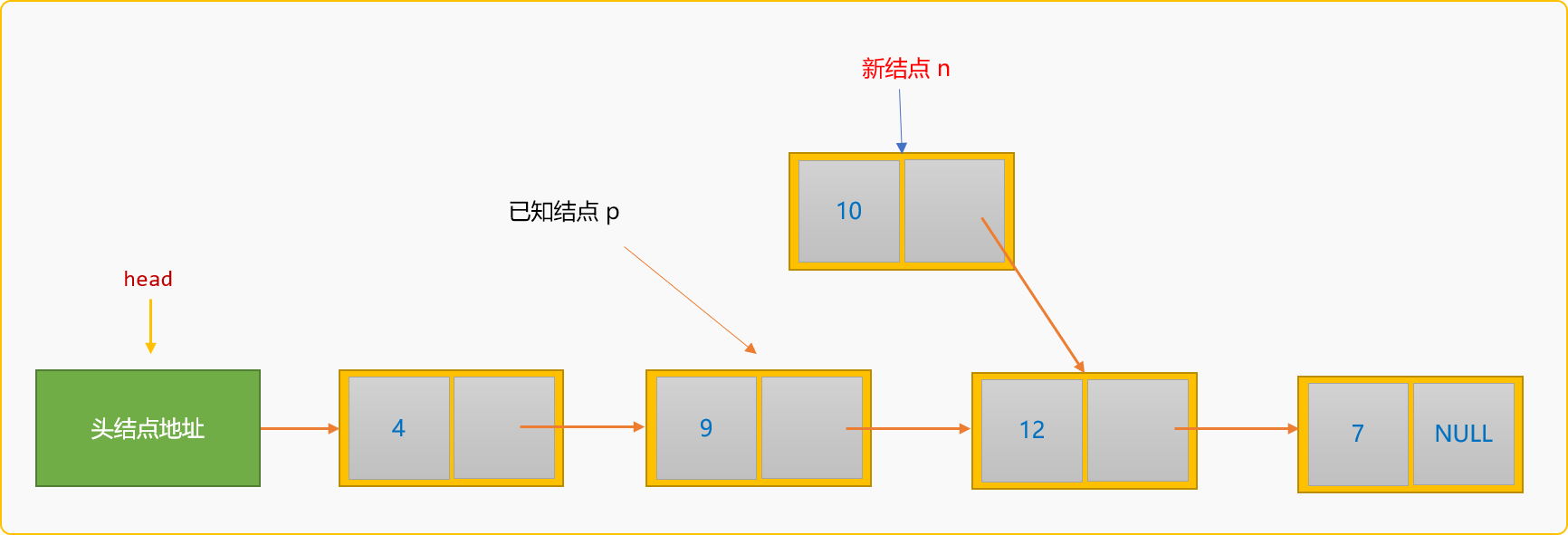• 指定`p`的后驱结点为新结点`n`

``````p->next=n;
``````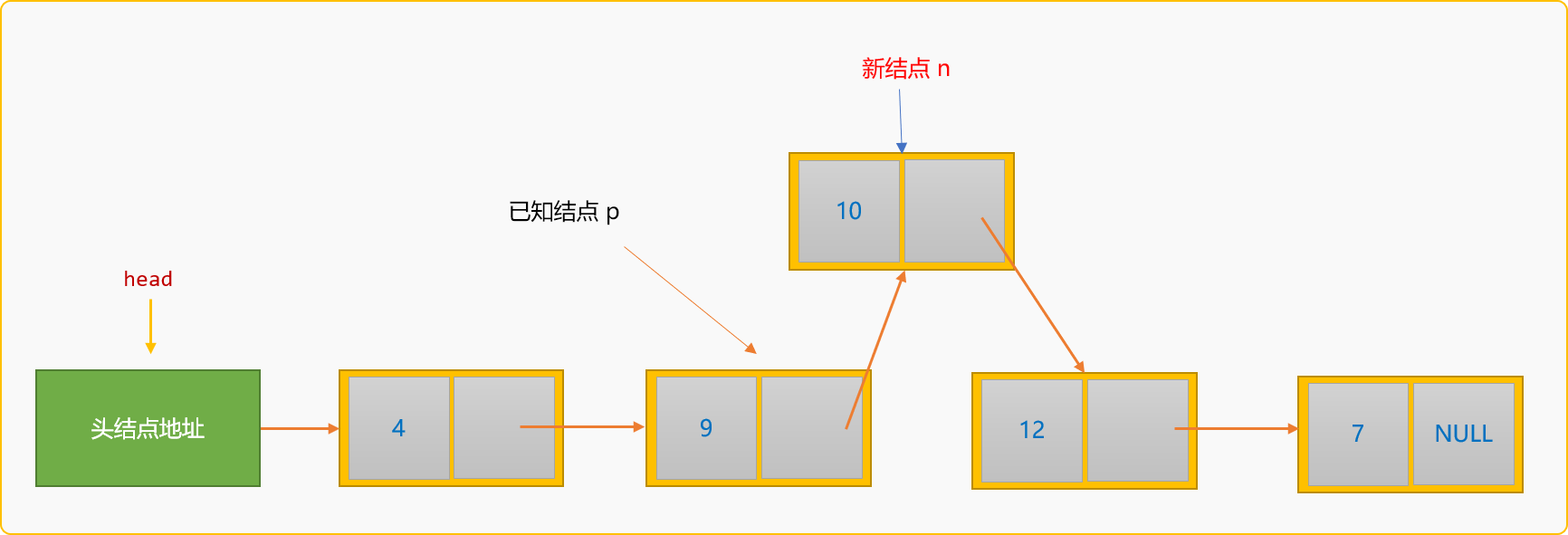``````//后插入
int instertAfter(dataType val,dataType data) {
//按值查找到结点
if (p==NULL){
return false;
}
n->data=data;
n->next=p->next;
p->next=n;
return true;
}
``````

``````int main(int argc, char** argv) {
//创建值为 1 ，2 ，3 的 3 个结点
list.createFromTail(3);
//在值为 2 的结点后面插入一个值为5 的新结点
list.instertAfter(2,5);
//在链表中查看是否存在值为 5 的结点
list.showSelf();
return 0;
}
``````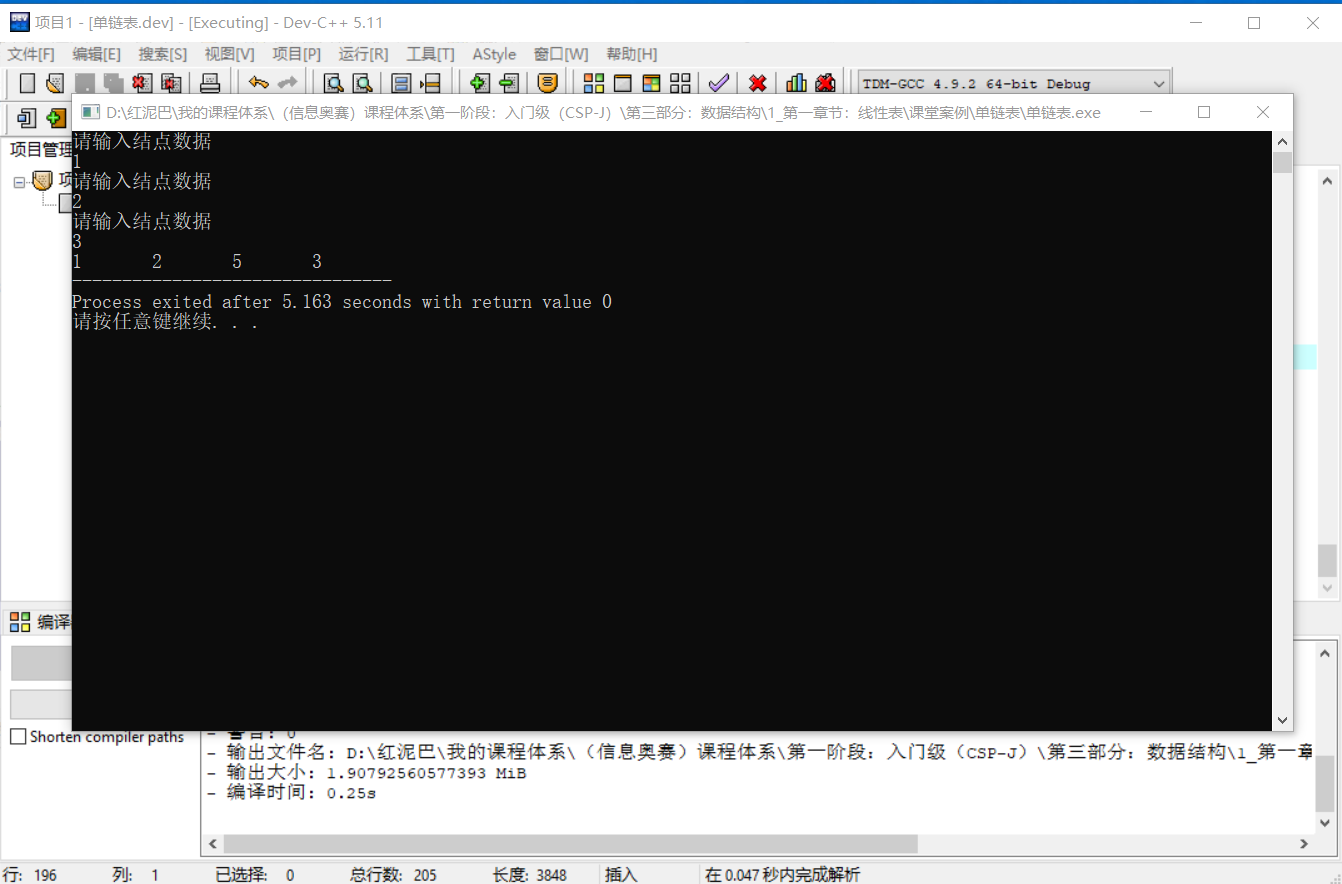#### 2.4.2 前插入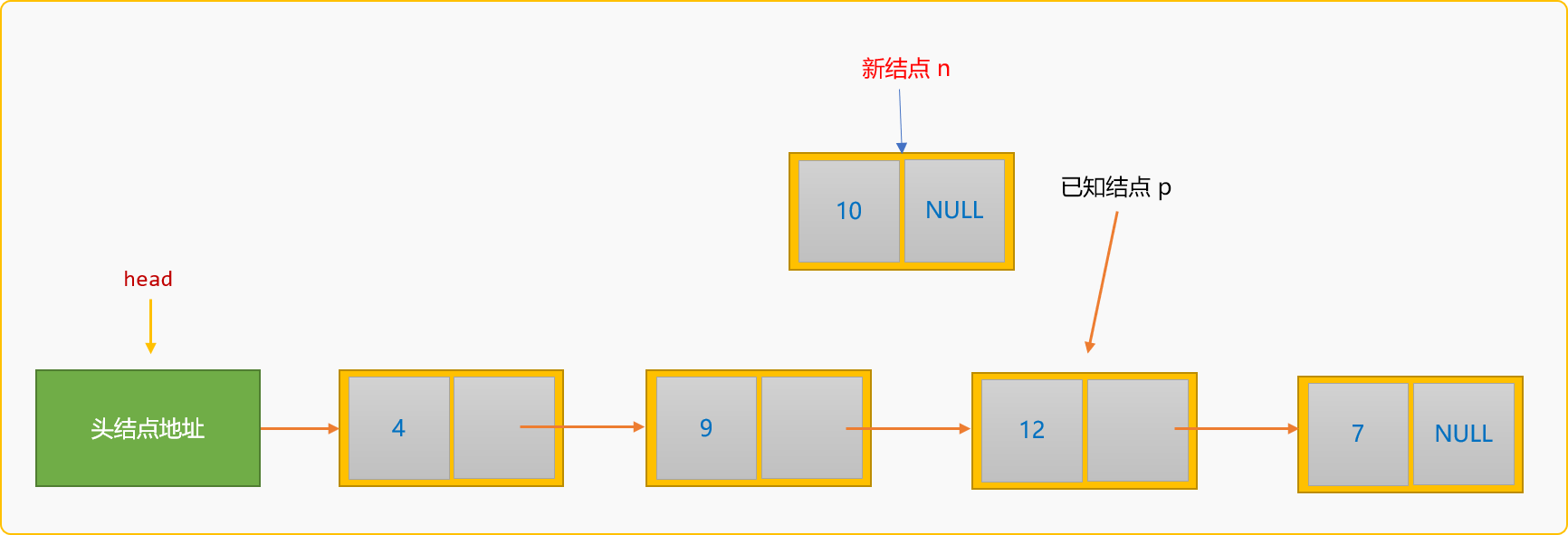• 除了要查找到`p`结点在链表中的地址，还需要知道`p`结点的前驱结点`p1`的地址。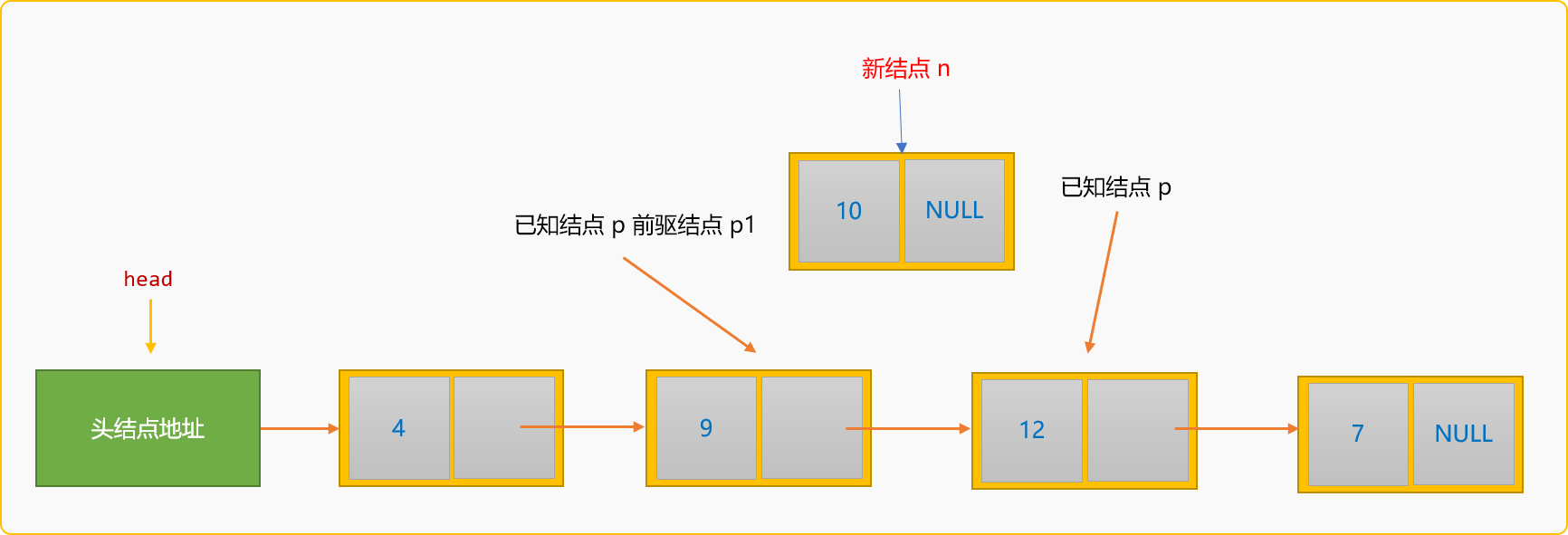• 创建新结点，然后指定新结点`n`的后驱结点为`p`结点。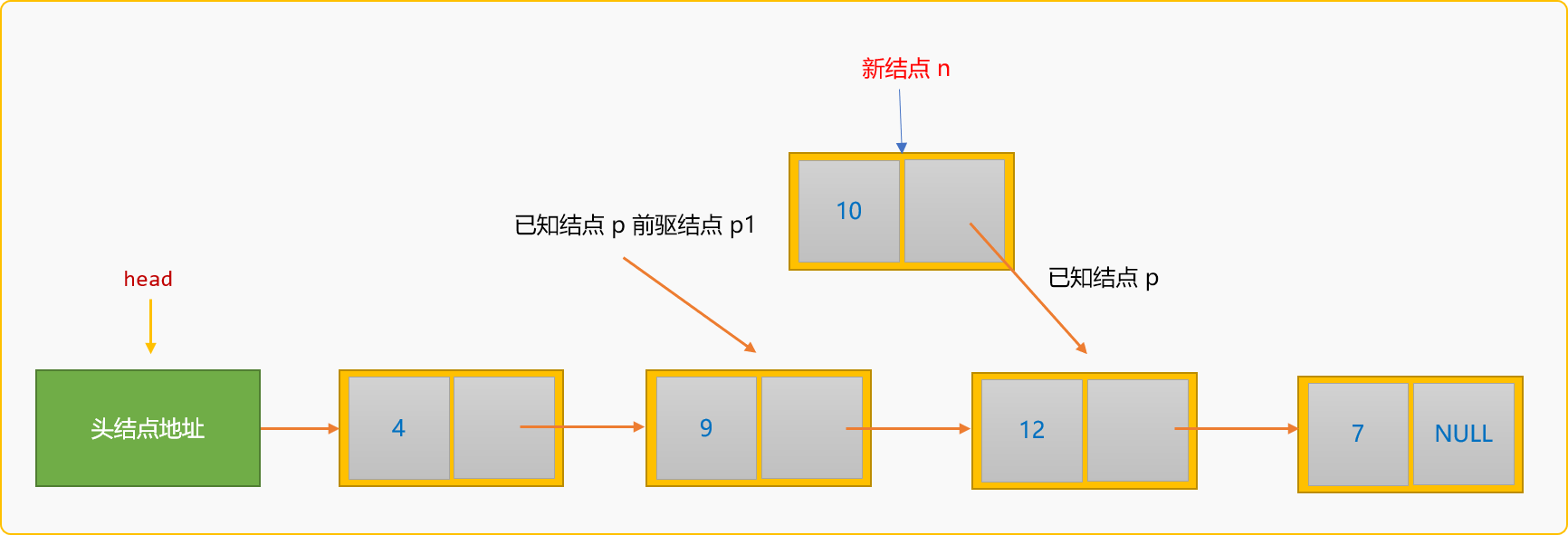• 指定结点`p1`的后驱结点为新结点`n`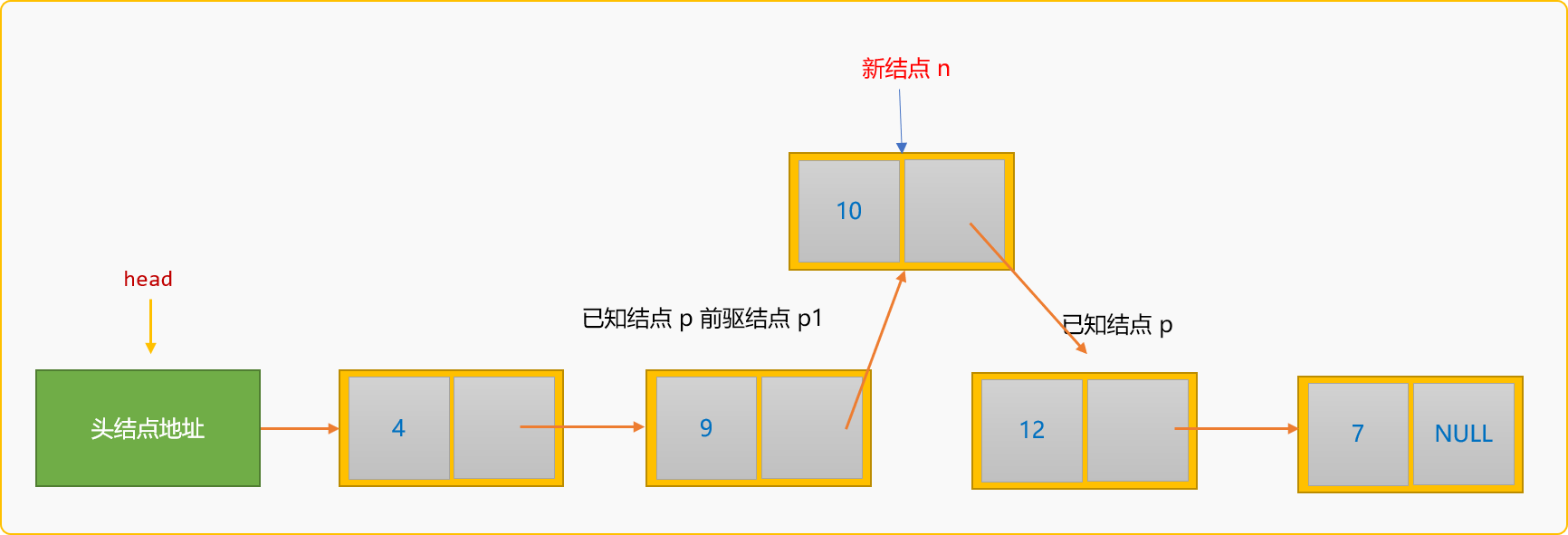``````/前插入
int insertBefore(dataType val,dataType data) {
//按值查找到结点
//查找前驱结点
while(p1->next!=p){
p1=p1->next;
}
//构建新结点
n->data=data;
//新结的点的后驱为 p 结点
n->next=p;
//重设 p1 的后驱为 n
p1->next=n;
}
``````

``````int main(int argc, char** argv) {
//创建值为 1 ，2 ，3 的 3 个结点
list.createFromTail(3);
//在值为 2 的结点前面插入一个值为5 的新结点
list.insertBefore(2,5);
//在链表中查看是否存在值为 5 的结点
list.showSelf();
return 0;
}
``````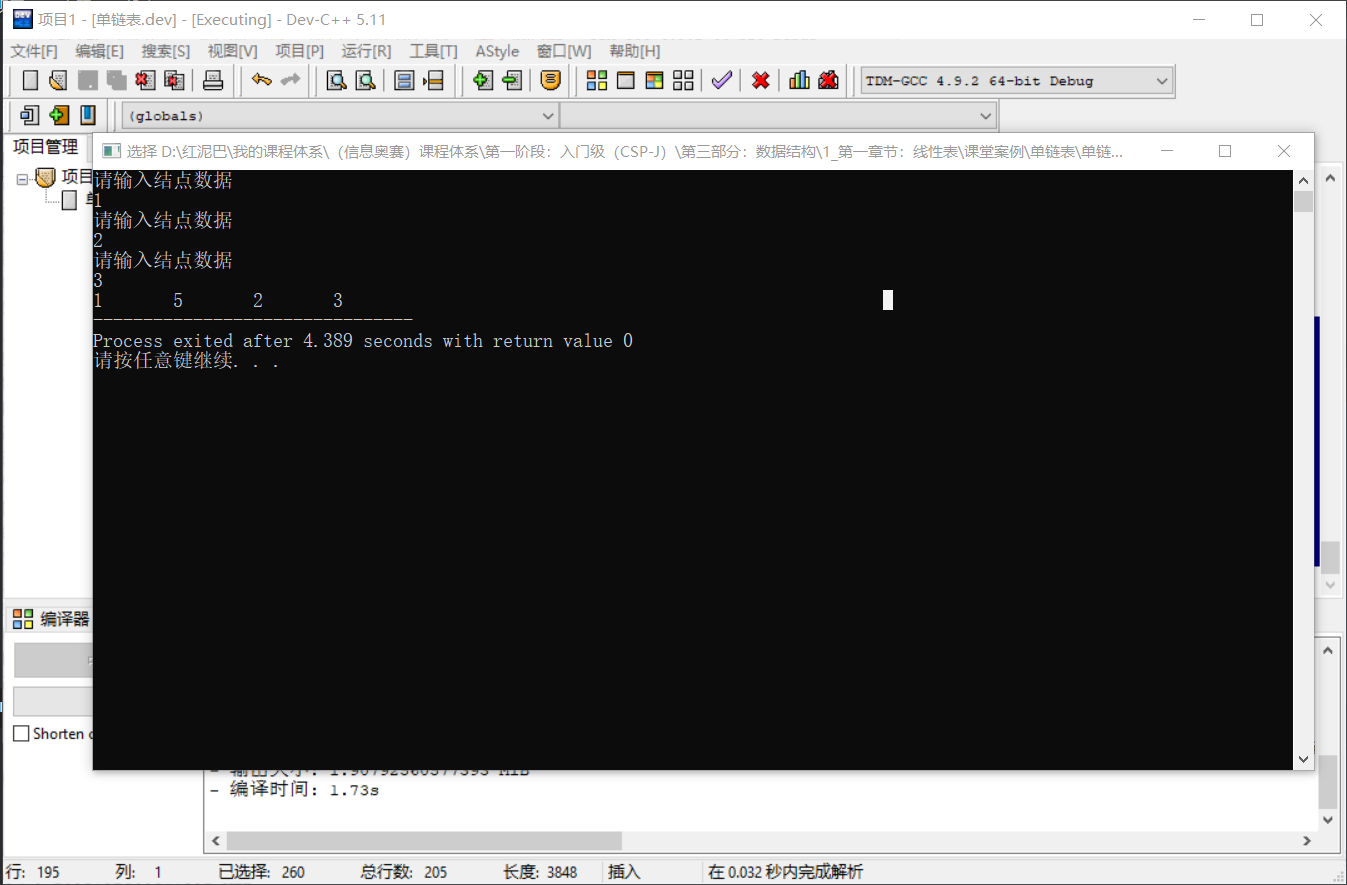### 2.5 删除

• 删除某一个结点。
• 删除所有结点，也称为清空链表。

#### 2.5.1 删除结点

• 在链表中查找到要删除的结点`p`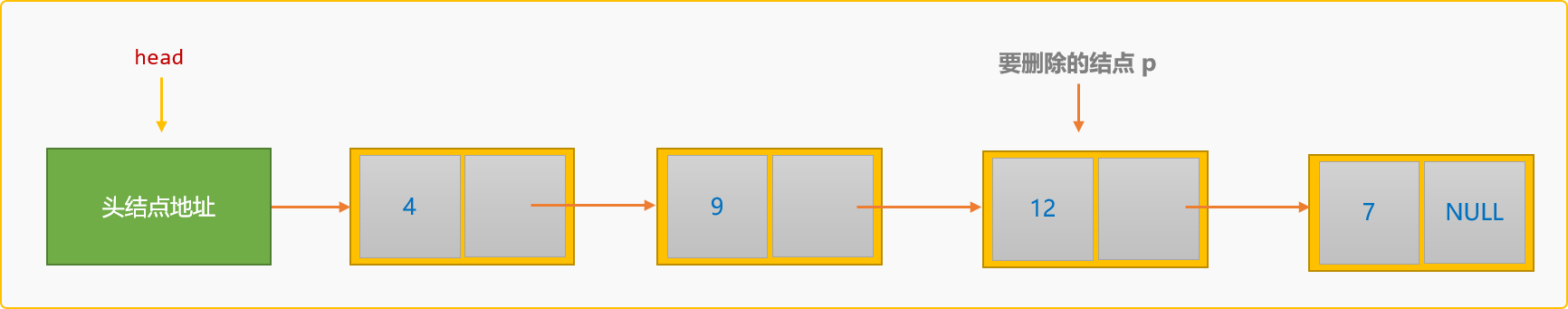• 找到`p`结点的前驱结点`p1`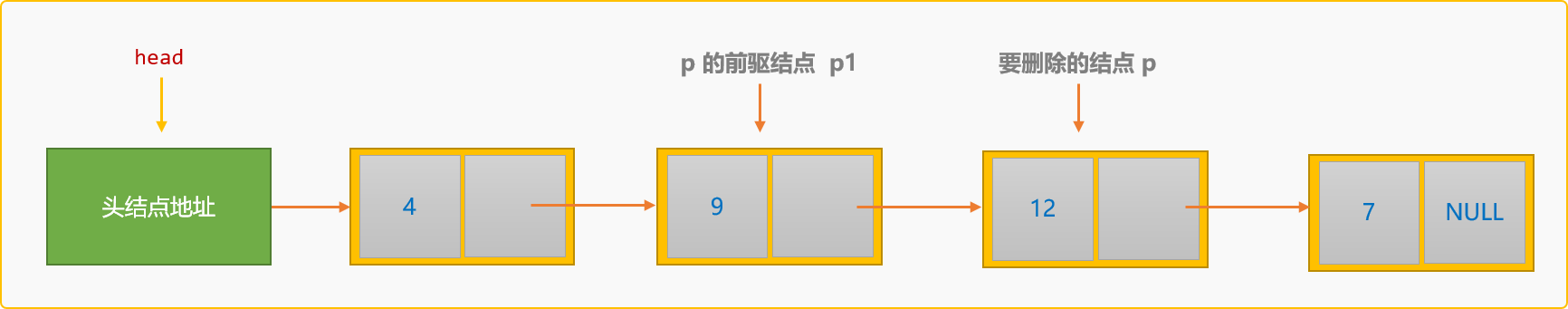• 指定 `p1`的后驱结点为 `p`的后驱结点。

``````p1->next=p->next;
``````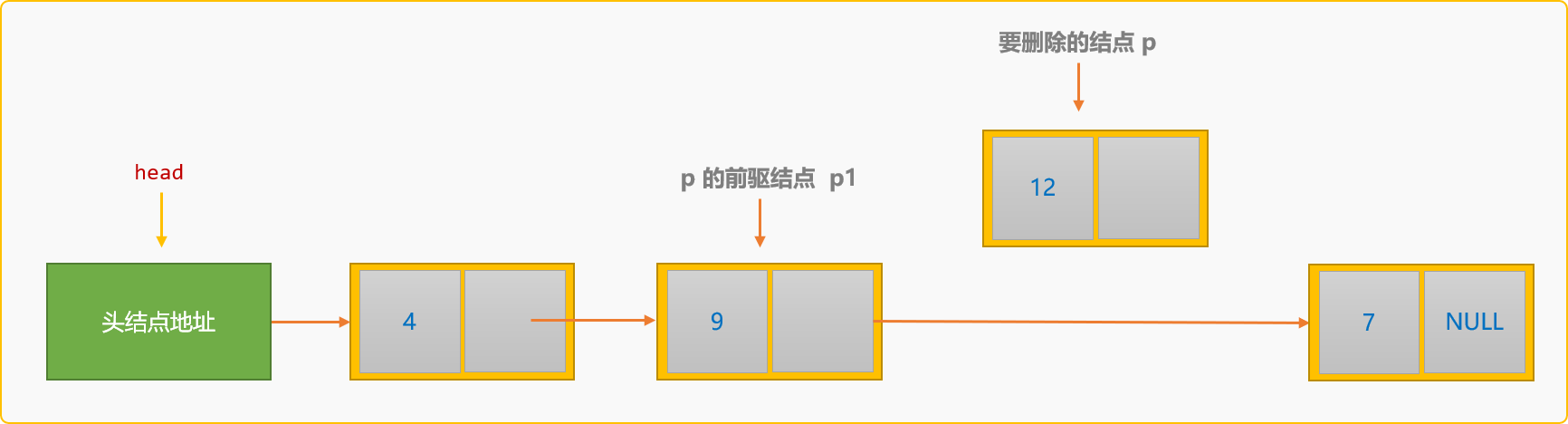``````//按值删除结点
int delNode(dataType data) {
//按值查找到要删除的结点
if (p==NULL)return false;
//查找前驱结点
while(p1->next!=p)p1=p1->next;
//删除操作
p1->next=p->next;
p->next=NULL;
delete p;
return true;
}
``````

``````int main(int argc, char** argv) {
//创建值为 1 ，2 ，3 的 3 个结点
list.createFromTail(3);
//删除值为 2 的结点
list.delNode(2);
list.showSelf();
return 0;
}
``````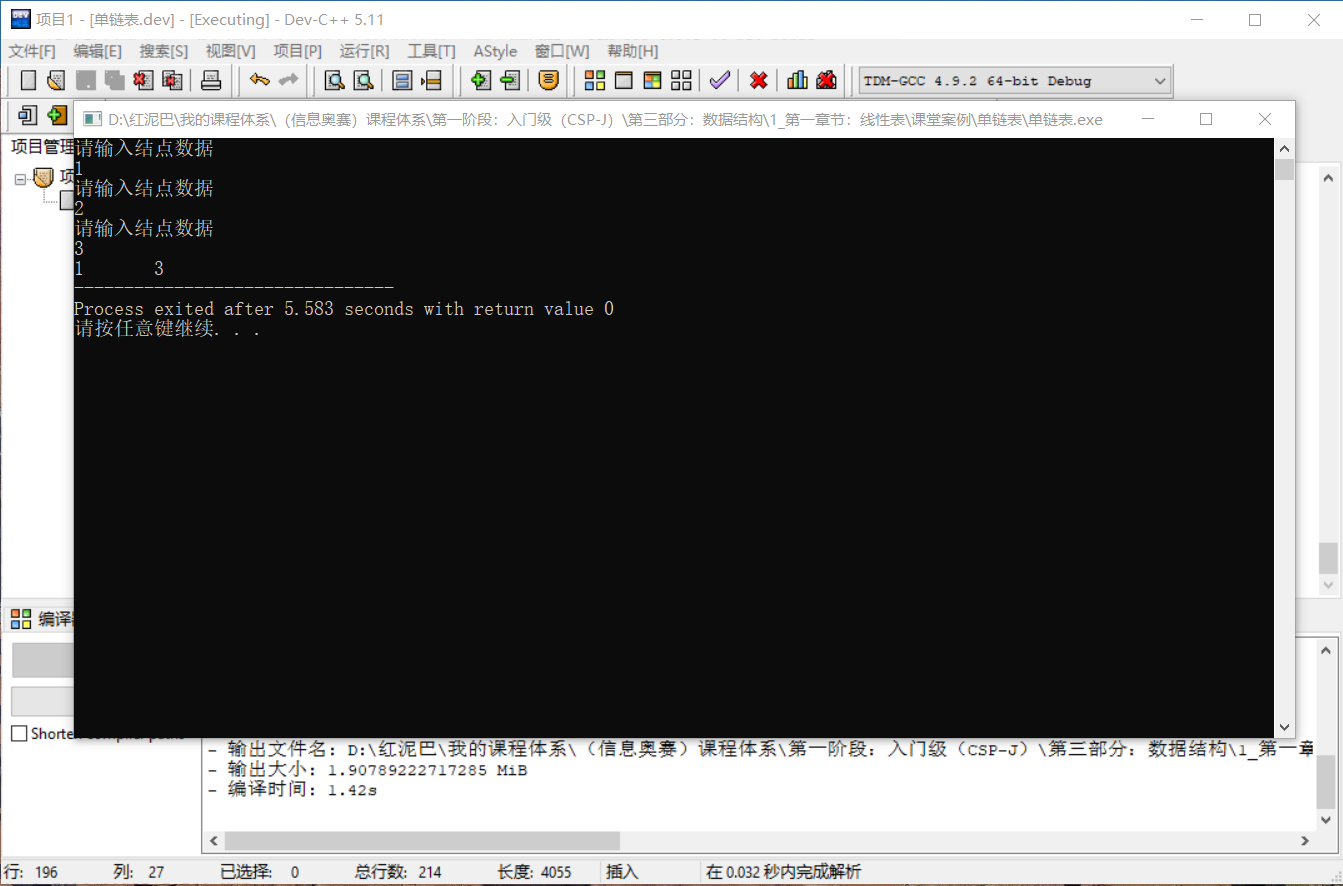#### 2.5.2 清空链表

``````void delAll() {
//临时结点
while(p!=NULL) {
//保留删除结点的后驱结点
q=p->next;
delete p;
p=q;
}
}
``````

``````int main(int argc, char** argv) {
//创建值为 1 ，2 ，3 的 3 个结点
list.createFromTail(3);
//没删除之前
cout<<"清除之前:"<<endl;
list.showSelf();
//清除链表
list.delAll();
//显示
cout<<endl<<"清除之后："<<endl;
list.showSelf();
return 0;
}
``````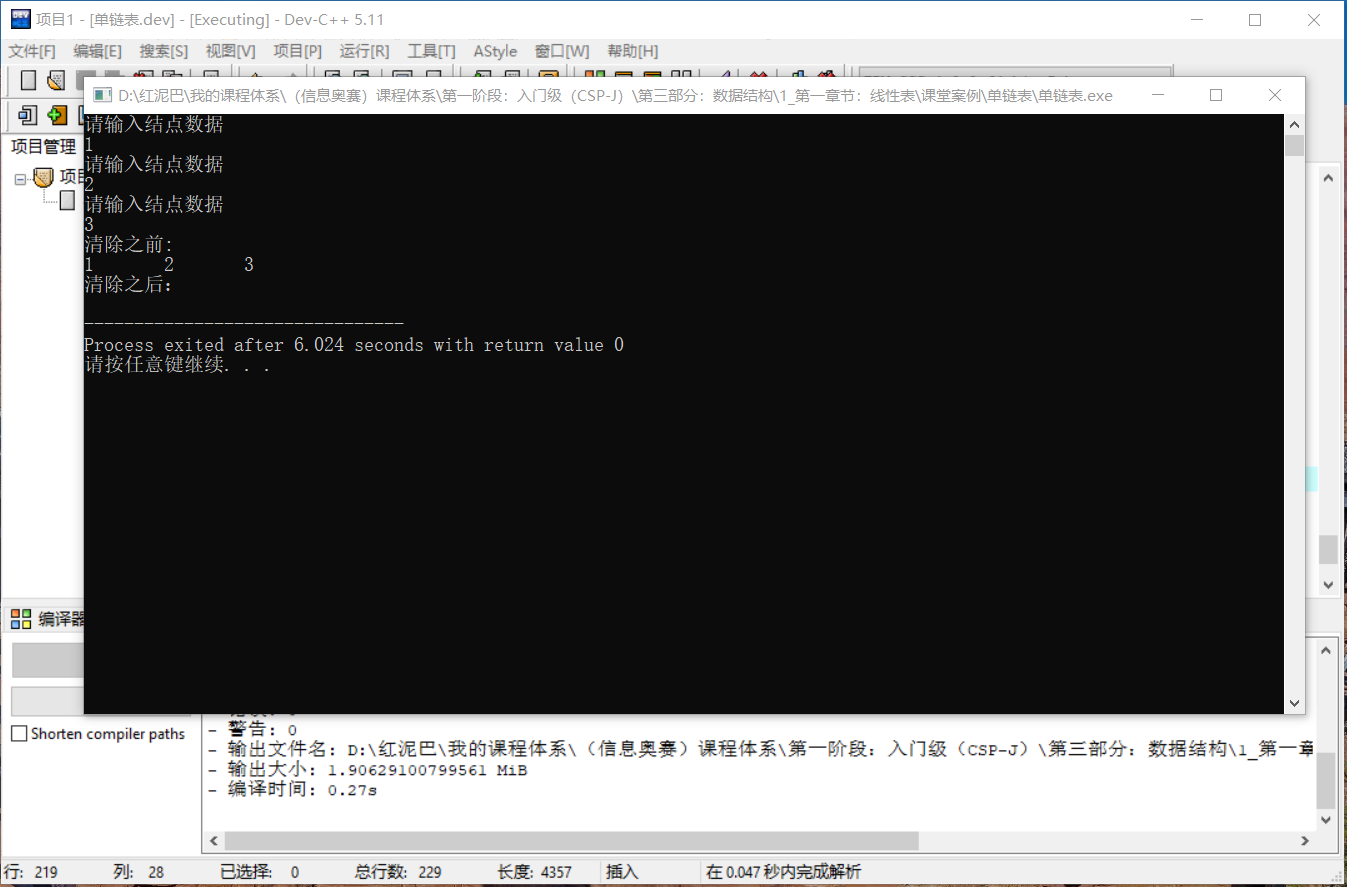### 2.6 时间复杂度分析

• 单链表上插入、删除一个结点，需要知道其前驱结点的地址。
• 单链表不具有按序号随机访问的特点，只能从头结点开始依次查询。